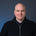# Animating a line#### Rory Creedon

04 Aug, 2016 04:14 PM

I have a quad line that I want to animate. Specifically the arc represents a flight path, and I want to animate that path, much like you would expect from a flight path, i.e. the curve is revealed as the hypothetical plane 'flies'.

I have tried a number of things in including resampling the line into 100 points and using frame to slice the list of points and passing that list to the line node. That did not work as expected.

Does anyone have any ideas?

1. Support Staff Posted by john on 05 Aug, 2016 05:33 AMHi Rory,

I figured out how to do this on a previous project.

The trick is to first Resample (I resample by length for consistent results), convert to a list of points, sort those points, then slice and reconnect. Sorting by x coordinate fixes the random resampling weirdness that occurs with some shapes and works well for west-to-east arcs; reverse the sort to fly the other direction.

I am attaching a demo network that draws the entire arc in gray, reveals a percentage of that arc in black, and also shows a marker moving along the arc. The Reveal subnetwork reveals a percentage of the arc using the above method. The Trace subnetwork provides the marker position; it uses a similar technique except that it returns a "point on path". You can either combine all three, or use the Reveal or Trace subnetworks independently.

You control the animation by changing the value of the number node from 0 to 100 - just feed in a frame node to create an MP4. Both subnetworks take a path as input; I used a random quadratic curve.

Also attaching screenshots of the overall network plus the Reveal subnetwork.

Hope that helps!

John

NOTE: For some reason I am unable to attach the NodeBox network file. I've tried everything I can think of without success. So here, in desperation, is the actual text of the NodeBox file. Please copy paste it into a text editor and save the resulting file with a .ndbx extension. NodeBox should be able to open it. Please reply and tell me if this worked:

<?xml version="1.0" encoding="UTF-8" standalone="no"?>
<ndbx formatVersion="21" type="file" uuid="5514cbdd-5998-408e-acc8-c492d9d4461f">
<property name="canvasHeight" value="1000"/>
<property name="canvasWidth" value="1000"/>
<property name="canvasX" value="0"/>
<property name="canvasY" value="0"/>
<node name="root" prototype="core.network" renderedChild="combine1">
<node name="colorize1" position="4.00,13.00" prototype="corevector.colorize">
<port name="fill" type="color" value="#00000000"/>
<port name="stroke" type="color" value="#bebebeff"/>
<port name="strokeWidth" type="float" value="3.0"/>
</node>
<node name="colorize2" position="10.00,13.00" prototype="corevector.colorize">
<port name="fill" type="color" value="#df0000ff"/>
</node>
<node name="colorize3" position="7.00,13.00" prototype="corevector.colorize">
<port name="fill" type="color" value="#00000000"/>
<port name="strokeWidth" type="float" value="3.0"/>
</node>
<node name="combine1" position="4.00,15.00" prototype="list.combine"/>
<node name="ellipse2" position="10.00,12.00" prototype="corevector.ellipse">
<port name="width" type="float" value="16.0"/>
<port name="height" type="float" value="16.0"/>
</node>
<node name="number1" position="8.00,7.00" prototype="math.number">
<port name="value" type="float" value="50.0"/>
</node>
<port name="point1" type="point" value="-177.00,42.00"/>
<port name="point2" type="point" value="236.00,-179.00"/>
<port name="distance" type="float" value="-124.0"/>
</node>
<node name="reveal" position="7.00,11.00" prototype="core.network" renderedChild="connect1">
<node name="connect1" position="76.00,19.00" prototype="corevector.connect">
<port name="closed" type="boolean" value="false"/>
</node>
<node position="81.00,10.00" prototype="math.convert_range">
<port name="target_end" type="float" value="0.99"/>
</node>
<node name="count1" position="77.00,14.00" prototype="list.count"/>
<node name="multiply1" position="80.00,16.00" prototype="math.multiply"/>
<node name="point1" position="76.00,11.00" prototype="corevector.point"/>
<node name="resample2" position="76.00,10.00" prototype="corevector.resample"/>
<node name="slice1" position="76.00,18.00" prototype="list.slice"/>
<node name="sort1" position="76.00,12.00" prototype="corevector.sort">
<port name="order_by" type="string" value="x"/>
</node>
<port childReference="resample2.shape" description="The input shape." label="" name="shape" range="value" type="geometry" widget="none"/>
<port childReference="convert_range.value" description="" label="" name="offset" range="value" type="float" value="50.0" widget="float"/>
<conn input="point1.value" output="resample2"/>
<conn input="sort1.shapes" output="point1"/>
<conn input="count1.list" output="sort1"/>
<conn input="slice1.size" output="multiply1"/>
<conn input="slice1.list" output="sort1"/>
<conn input="connect1.points" output="slice1"/>
<conn input="multiply1.value1" output="count1"/>
<conn input="multiply1.value2" output="convert_range"/>
</node>
<node name="trace" position="10.00,11.00" prototype="core.network" renderedChild="point_on_path">
<node name="connect1" position="53.00,-1.00" prototype="corevector.connect">
<port name="closed" type="boolean" value="false"/>
</node>
<node position="57.00,-4.00" prototype="math.convert_range">
<port name="target_end" type="float" value="99.9"/>
</node>
<node name="point1" position="53.00,-3.00" prototype="corevector.point"/>
<node position="56.00,1.00" prototype="corevector.point_on_path">
<port name="t" type="float" value="58.0"/>
</node>
<node name="resample2" position="53.00,-4.00" prototype="corevector.resample"/>
<node name="sort1" position="53.00,-2.00" prototype="corevector.sort">
<port name="order_by" type="string" value="x"/>
</node>
<port childReference="resample2.shape" description="The input shape." label="" name="shape" range="value" type="geometry" widget="none"/>
<port childReference="convert_range.value" description="" label="" name="offset" range="value" type="float" value="50.0" widget="float"/>
<conn input="point1.value" output="resample2"/>
<conn input="sort1.shapes" output="point1"/>
<conn input="connect1.points" output="sort1"/>
<conn input="point_on_path.shape" output="connect1"/>
<conn input="point_on_path.t" output="convert_range"/>
</node>
<conn input="combine1.list1" output="colorize1"/>
<conn input="colorize2.shape" output="ellipse2"/>
<conn input="combine1.list7" output="colorize2"/>
<conn input="ellipse2.position" output="trace"/>
<conn input="trace.offset" output="number1"/>
<conn input="reveal.offset" output="number1"/>
<conn input="colorize3.shape" output="reveal"/>
<conn input="combine1.list3" output="colorize3"/>
</node>
</ndbx>

2. Support Staff Posted by john on 08 Aug, 2016 03:27 AMFrederik suggested attaching a zip of the ndbx file. Let's see it that works...

3. Posted by Rory Creedon on 10 Aug, 2016 02:54 PMHAHAH, this is totally brilliant!

I am going to enjoy playing with this, I may revert with some questions as I reverse engineer this. thank yoU!

4. Posted by andy.treichler on 17 Aug, 2017 03:49 AMNice feature! I like it :-)

Formatting help / Preview (switch to plain text) No formatting (switch to Markdown)

### »

#### Attached Files

You can attach files up to 10MB

If you don't have an account yet, we need to confirm you're human and not a machine trying to post spam.

# Keyboard shortcuts

### Generic

? Show this help Blurs the current field

### Comment Form

r Focus the comment reply box Submit the comment

You can use `Command ⌘` instead of `Control ^` on Mac

## Recent Discussions

 06 Sep, 2023 07:20 AM Trying to create a Sankey diagram 05 Sep, 2023 08:40 PM Quadtree Node 30 Aug, 2023 03:16 AM Mesh Node 19 Aug, 2023 03:22 AM Scatter Bias Node 29 Jul, 2023 05:52 AM Ramp Nodes
 28 Jul, 2023 10:53 PM Combinatoric Toolkit 23 Jul, 2023 07:04 AM timeline rough (very rough) 14 Jul, 2023 03:05 AM The Black Lavender Square Mosaic 07 Jul, 2023 06:26 AM How to get the type of an item 05 Jul, 2023 11:44 PM Yin Yang Challenge 30 Jun, 2023 12:48 PM NodeBox with JS ( D3.JS or P5.JS ) For FxHash